# TF-IDF and Cosine Similarity in Machine Learning

## TF-IDF and Cosine Similarity in Machine Learning

In this article, I am going to discuss TF-IDF and Cosine Similarity in Machine Learning and their application to Vector Space Model with Examples. Please read our previous article where we discussed K-Means Clustering in Machine Learning with Examples.

##### TF-IDF in Machine Learning

Term Frequency is abbreviated as TF-IDF. Records with an inverse Document Frequency. It’s the process of determining how relevant a word in a series or corpus is to a text. The meaning of a word grows in proportion to how many times it appears in the text, but this is offset by the corpus’s word frequency (data-set).

##### Term Frequency in Machine Learning

The frequency in document d represents the number of times a certain word t appears. As a result, we can observe that when a term appears in the text, it gets more relevant, which is reasonable. We can use a vector to describe the text in the bag of word models because the ordering of terms isn’t important.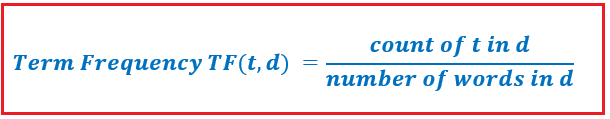There is an entry for each individual phrase in the document, with the value being the term frequency. The weight of a term in a document is simply proportional to the frequency of the term.

##### Inverse Document Frequency in Machine Learning

It is a term that refers to the frequency with It mostly assesses the word’s relevance. The main goal of the search is to find relevant records that match the requirement. Because tf considers all terms to be equally meaningful, the term frequencies cannot be used to determine the weight of a term in the document. Here’s how we can calculate the inverse document frequency:Tf-idf is one of the strongest metrics for determining the importance of a term in a series or corpus of texts. The tf-idf weighting scheme gives each word in a document a weight based on its term frequency (tf) and inverse document frequency (idf). Words with higher weight ratings are considered to be more significant.

The tf-idf weight is usually made up of two terms:

1. Normalized Term Frequency (tf)
2. Inverse Document Frequency (idf)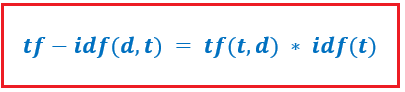Let’s consider this example –

1. She loves food with cheese.
2. Her favorite food is Italian.
3. She lives in the Italian state.

Let’s see what the normalized term frequency will look like –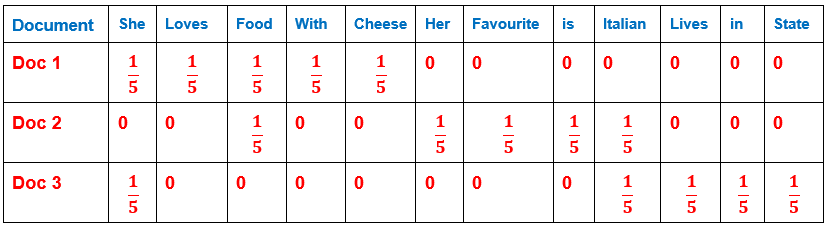Now, let’s see the IDF values for these terms –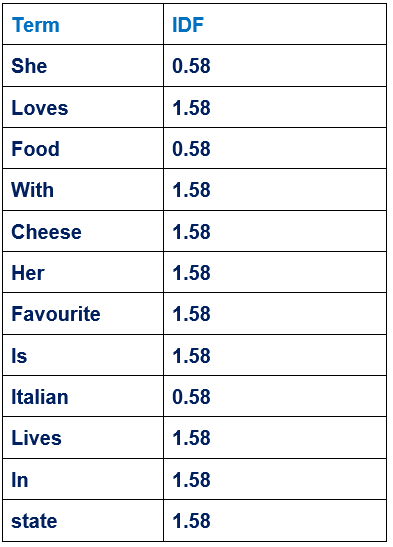TF-IDF values for all the terms in respective documents –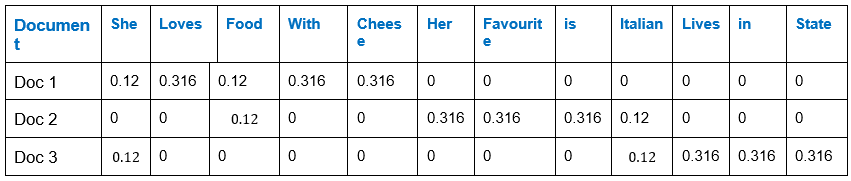##### Cosine Similarity in Machine Learning

The cosine similarity between two vectors (or two documents in Vector Space) is a statistic that estimates the cosine of their angle. Because we’re not only considering the magnitude of each word count (tf-idf) of each text, but also the angle between the documents, this metric can be considered as a comparison between documents on a normalized space. This is the formula for cosine similarity –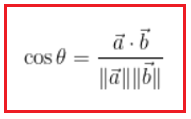Cosine Similarity generates a measure that indicates how closely two documents are connected by looking at the angle rather than the magnitude, as seen in the examples below: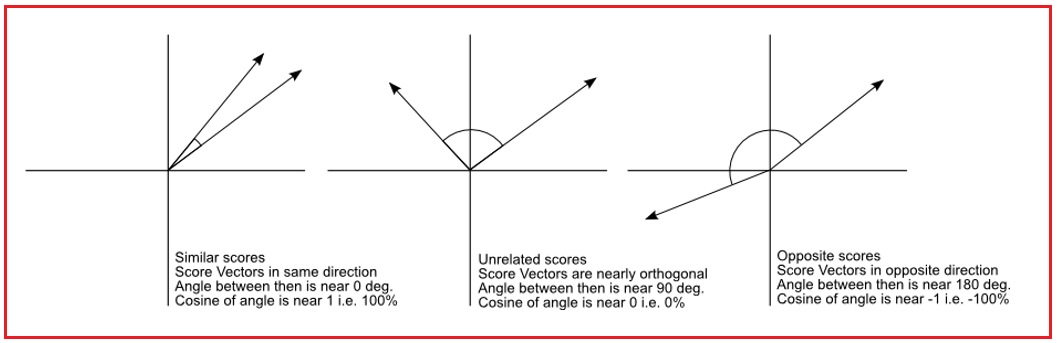The central issue in the application of Cosine Similarity is that the measurement tends to overlook the greater term count on documents, even if we have a vector going to a position remote from another vector. If a paper has the term “sky” 200 times and another contains the word “sky” 50 times, the Euclidean distance between them will be greater, but the angle will still be tiny because they are pointing in the same direction, which is what matters when comparing documents.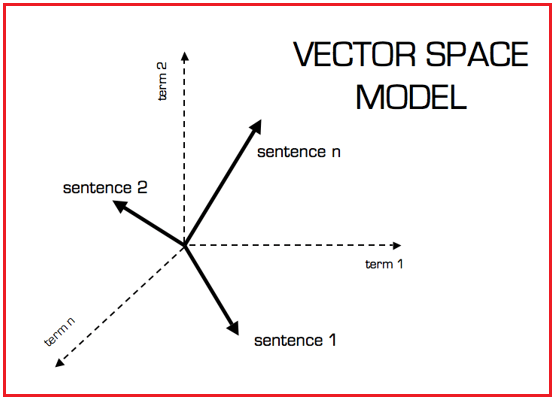We can use scikit-learn to do it in practice now that we have a Vector Space Model of documents characterized as vectors (with TF-IDF counts) and a formula to calculate the similarity between distinct documents in this space (sklearn).

#### Case Study in E-Commerce

##### Problem Statement

Womava is an American online women’s apparel brand that designs and sells a variety of styles for women. They’re looking for the most cost-effective solution that will increase their revenue while lowering their advertising costs. They’ve amassed a database that revolves around client reviews. They employed a team of data scientists to fix the problem.

##### Importing Libraries
```import pandas as pd
import numpy as np
from afinn import Afinn
import plotly.graph_objs as go
from sklearn.preprocessing import StandardScaler
from sklearn.cluster import KMeans

print('Shape of the dataset:', data.shape)

```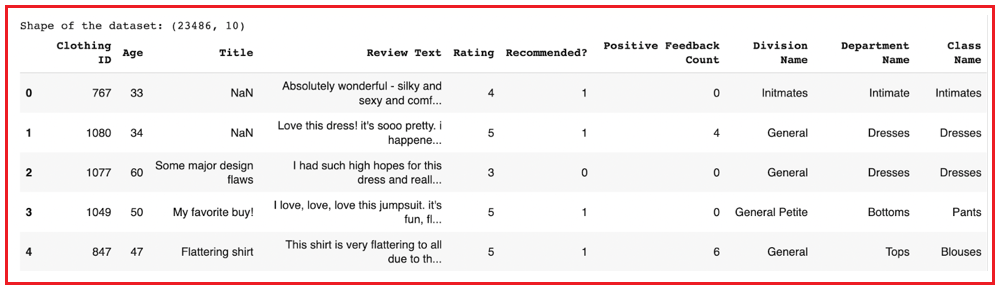##### Data Description

In this section, we will get information about the data and see some observations.

data.describe()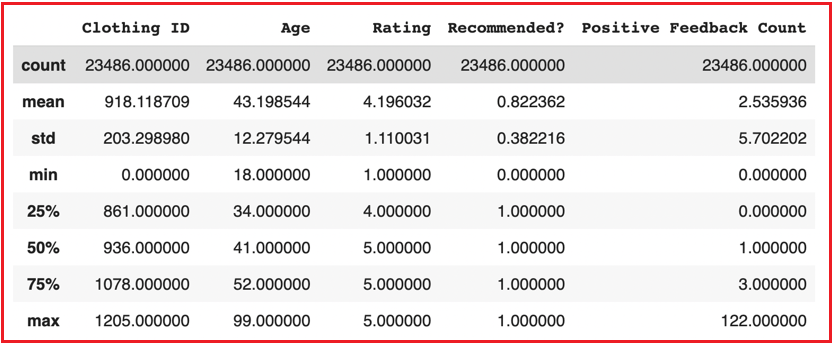data.info()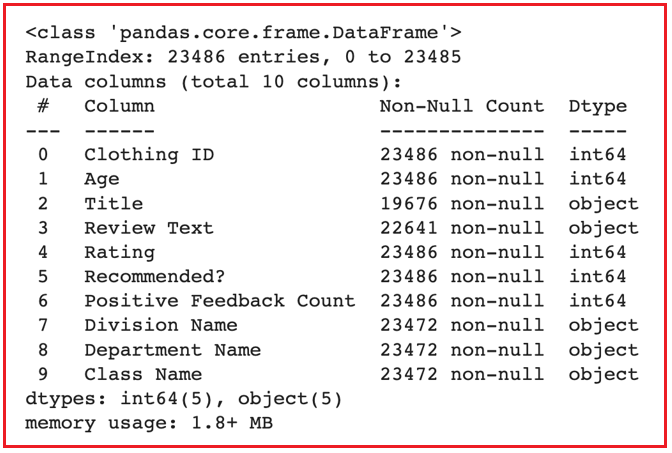##### Data Pre-Processing
```# Drop cells from Title and Review Text
data.dropna(subset=['Title', 'Review Text'], inplace=True)

# Replace Division Name, Department Name, and Class Name with mode values
data['Division Name'] = data['Division Name'].replace(np.nan, data['Division Name'].mode())
data['Department Name'] = data['Department Name'].replace(np.nan, data['Department Name'].mode())
data['Class Name'] = data['Class Name'].replace(np.nan, data['Class Name'].mode())

# Initiating the affin object
afinn = Afinn()

# Transforming the Review text to lower letters
data['Review Text'] = data['Review Text'].str.lower()

# Generate sentiment scores using the Review Text
data['Sentiment Score'] = data.apply(lambda row: afinn.score(row['Review Text']), axis=1)
```
##### Q. What is the distribution of the Age features?
```# Extract labels and values of menu category
labels = data['Age'].value_counts().index
values = data['Age'].value_counts().values

# Initiate an empty figure
fig = go.Figure()

# Add a trace of bar to the figure

# Update the layout with some cosmetics
fig.update_layout(height=500,
width=1000,
title_text='Frequency Distribution of Age',
title_x=0.5,
yaxis_title='Frequency',
xaxis_title='Age')

# Display the figure
fig.show()
```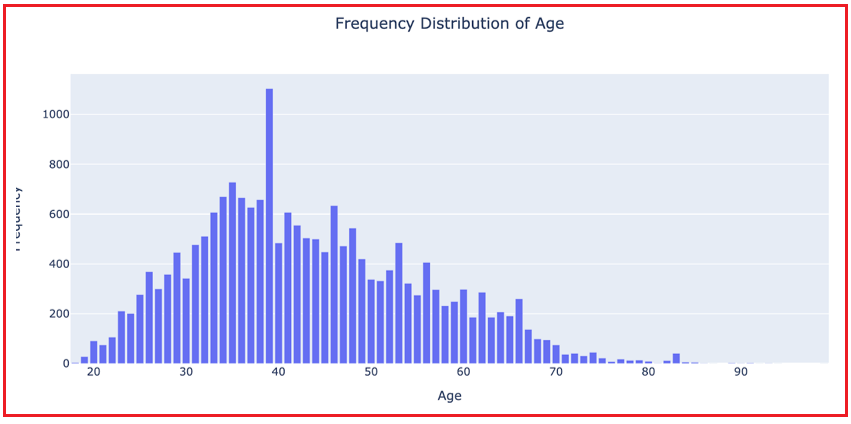##### Q. What is the frequency distribution of Sentiment Scores towards clothing?
```# Extract labels and values of positive sentiments
positivelabels = data[data['Sentiment Score'] > 0]['Sentiment Score'].value_counts().index
positivevalues = data[data['Sentiment Score'] > 0]['Sentiment Score'].value_counts().values

# Extract labels and values of neutral sentiments
neutrallabels = data[data['Sentiment Score'] == 0]['Sentiment Score'].value_counts().index
neutralvalues = data[data['Sentiment Score'] == 0]['Sentiment Score'].shape

# Extract labels and values of negative sentiments
negativelabels = data[data['Sentiment Score'] < 0]['Sentiment Score'].value_counts().index
negativevalues = data[data['Sentiment Score'] < 0]['Sentiment Score'].value_counts().values

# Initiate an empty figure
fig = go.Figure()

# Adding a trace of positive sentiments
x=positivelabels,
width=0.4,
marker_color='#34A853',
name='Positive Sentiments'))

# Adding a trace of neutral sentiments
x=neutrallabels,
width=0.4,
marker_color='#35363A',
name='Neutral Sentiments'))

# Adding a trace of negative sentiments
x=negativelabels,
width=0.4,
marker_color='#ed7d31',
name='Negative Sentiments'))

# Update the layout with some cosmetics
fig.update_layout(height=500,
width=1200,
title_text='Freuqency Distribution of Sentiment Scores',
title_x=0.5,
xaxis_title='Score Label',
yaxis_title='Frequency')

# Display the figure
fig.show()
```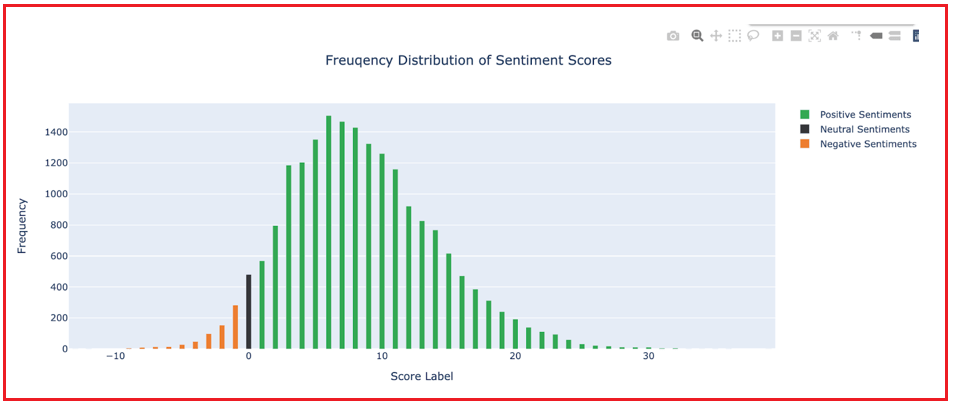```datax = data[['Age', 'Sentiment Score']]

# Log transformation to handle positive skewness
datax['Age'] = np.log(datax['Age'])

# Scaling the Age and Sentiment Scores features
scaler = StandardScaler()
scaled_data = scaler.fit_transform(datax)
scaled_frame = pd.DataFrame(data=scaled_data, columns=datax.columns)
```
##### Model Building
```inertia_vals = []
K_vals = [x for x in range(1, 16)]

for i in K_vals:
k_model = KMeans(n_clusters=i, max_iter=500, random_state=42, n_jobs=-1)
k_model.fit(scaled_frame)
inertia_vals.append(k_model.inertia_)

# Visualzing the Inertia vs K Values
fig = go.Figure()

fig.add_trace(go.Scatter(x = K_vals, y = inertia_vals, mode = 'lines+markers'))
fig.update_layout(xaxis = dict(tickmode = 'linear', tick0 = 1, dtick = 1),
title_text = 'Within Cluster Sum of Squared Distances VS K Values',
title_x = 0.5,
xaxis_title = 'K values',
yaxis_title = 'Cluster Sum of Squared Distances')
fig.show()
```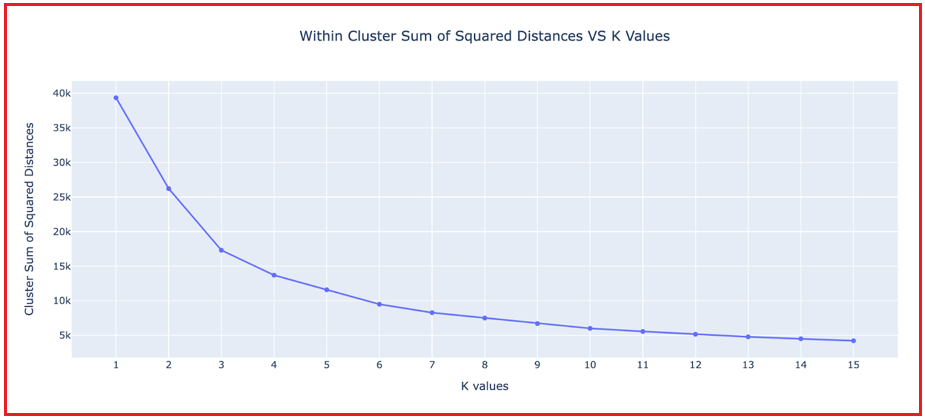```kmeans = KMeans(n_clusters=6, max_iter=500, random_state=42, n_jobs=-1)
kmeans.fit(X=scaled_frame)
scaled_frame['Labels'] = kmeans.labels_
centers = kmeans.cluster_centers_

# Initiate an empty figure
fig = go.Figure()

# Add a first trace of scatter to the figure
y=scaled_frame['Sentiment Score'],
text=scaled_frame.index,
name='',
mode='markers',
marker=go.Marker(sizemode='diameter',
opacity=0.5,
color=scaled_frame['Labels']),
showlegend=False))
# Add a second trace of scatter (centers) to the figure
y=centers[:, 1],
text=['Cluster '+ str(i) for i in range(6)],
name='',
mode='markers',
marker=go.Marker(symbol='x',
size=12,
color='rgba(66, 5, 84, 1.0)'),
showlegend=False))

# Update the layout with some cosmetics
fig.update_layout(height=500,
width=1000,
title_text='Visualization of Clustered Data',
title_x=0.5,
xaxis_title='Feature Space 1 (Age)',
yaxis_title='Feature Space 2 (Sentiment Score)')

# Display the figure
fig.show()
```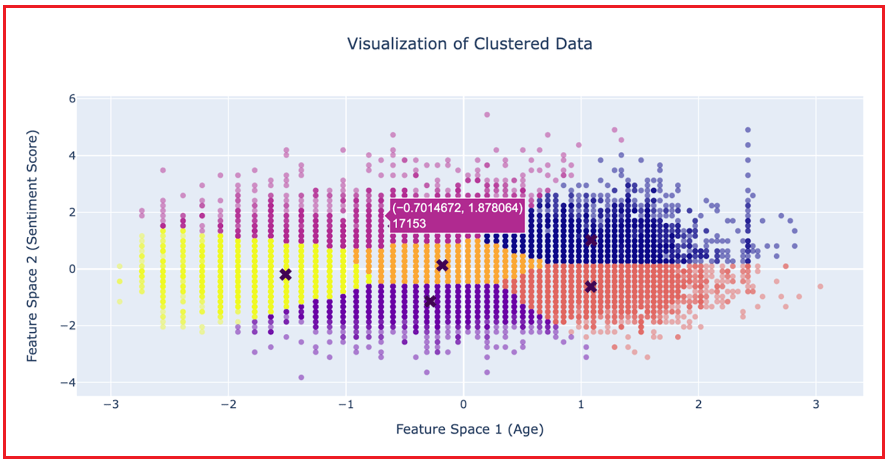In the next article, I am going to discuss Association Rules and their Use Cases in Machine Learning with Examples. Here, in this article, I try to explain TF-IDF and Cosine Similarity in Machine Learning and their application to Vector Space Model with Examples. I hope you enjoy this TF-IDF and Cosine Similarity in Machine Learning with Examples article.